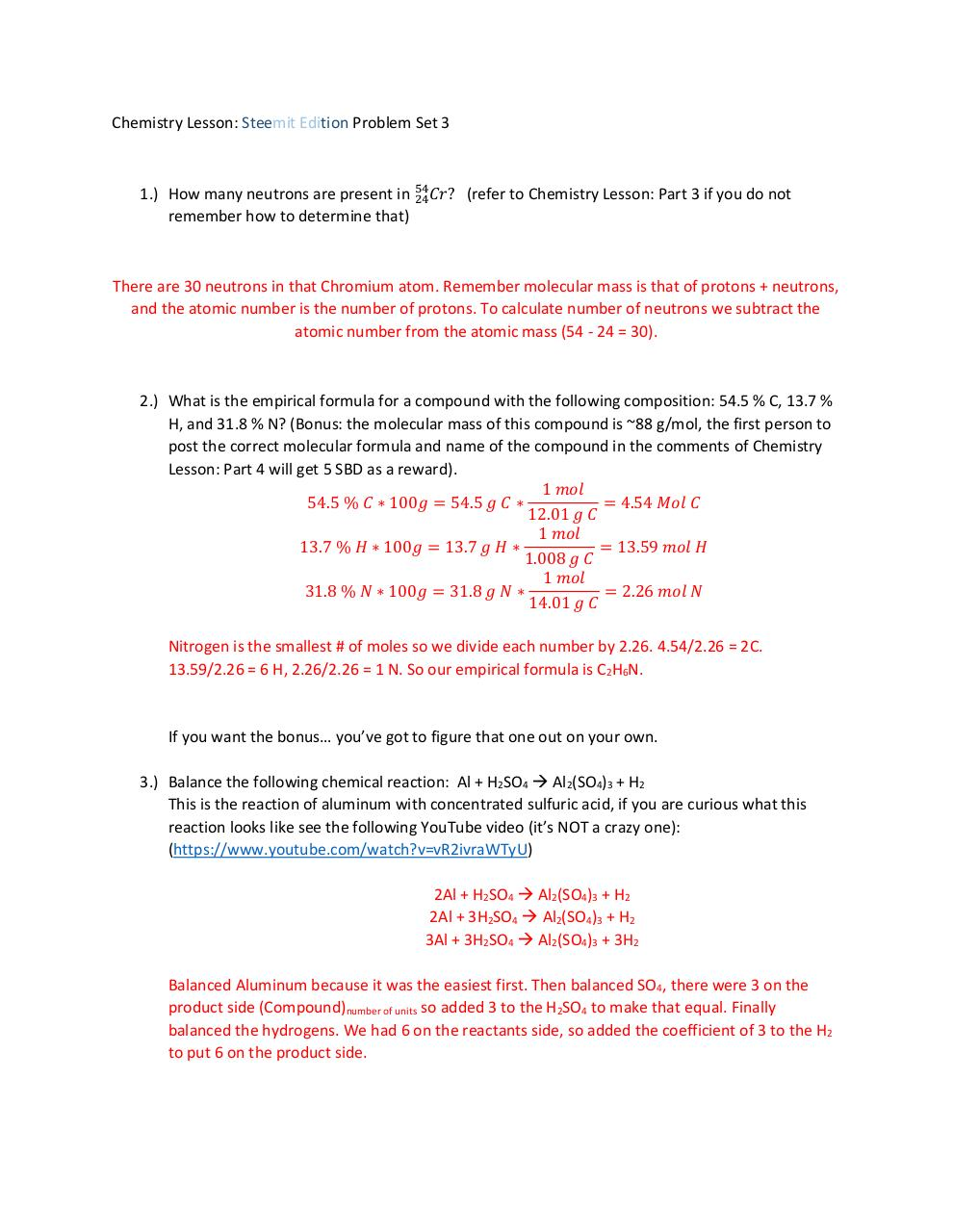# Problem Set 3 Answer Key .pdf

### File information

Original filename: Problem Set 3 Answer Key.pdf
Author: Robert Bauer

This PDF 1.5 document has been generated by MicrosoftÂ® Word 2013, and has been sent on pdf-archive.com on 10/09/2016 at 03:09, from IP address 50.164.x.x. The current document download page has been viewed 996 times.
File size: 272 KB (1 page).
Privacy: public file

Problem Set 3 Answer Key.pdf (PDF, 272 KB)

### Document preview

Chemistry Lesson: Steemit Edition Problem Set 3

1.) How many neutrons are present in 54
24𝐶𝑟 ? (refer to Chemistry Lesson: Part 3 if you do not
remember how to determine that)

There are 30 neutrons in that Chromium atom. Remember molecular mass is that of protons + neutrons,
and the atomic number is the number of protons. To calculate number of neutrons we subtract the
atomic number from the atomic mass (54 - 24 = 30).

2.) What is the empirical formula for a compound with the following composition: 54.5 % C, 13.7 %
H, and 31.8 % N? (Bonus: the molecular mass of this compound is ~88 g/mol, the first person to
post the correct molecular formula and name of the compound in the comments of Chemistry
Lesson: Part 4 will get 5 SBD as a reward).
1 𝑚𝑜𝑙
54.5 % 𝐶 ∗ 100𝑔 = 54.5 𝑔 𝐶 ∗
= 4.54 𝑀𝑜𝑙 𝐶
12.01 𝑔 𝐶
1 𝑚𝑜𝑙
13.7 % 𝐻 ∗ 100𝑔 = 13.7 𝑔 𝐻 ∗
= 13.59 𝑚𝑜𝑙 𝐻
1.008 𝑔 𝐶
1 𝑚𝑜𝑙
31.8 % 𝑁 ∗ 100𝑔 = 31.8 𝑔 𝑁 ∗
= 2.26 𝑚𝑜𝑙 𝑁
14.01 𝑔 𝐶
Nitrogen is the smallest # of moles so we divide each number by 2.26. 4.54/2.26 = 2C.
13.59/2.26 = 6 H, 2.26/2.26 = 1 N. So our empirical formula is C2H6N.

If you want the bonus… you’ve got to figure that one out on your own.
3.) Balance the following chemical reaction: Al + H2SO4  Al2(SO4)3 + H2
This is the reaction of aluminum with concentrated sulfuric acid, if you are curious what this
reaction looks like see the following YouTube video (it’s NOT a crazy one):
2Al + H2SO4  Al2(SO4)3 + H2
2Al + 3H2SO4  Al2(SO4)3 + H2
3Al + 3H2SO4  Al2(SO4)3 + 3H2
Balanced Aluminum because it was the easiest first. Then balanced SO4, there were 3 on the
product side (Compound)number of units so added 3 to the H2SO4 to make that equal. Finally
balanced the hydrogens. We had 6 on the reactants side, so added the coefficient of 3 to the H2
to put 6 on the product side.#### HTML Code

Copy the following HTML code to share your document on a Website or Blog

#### QR Code### Related keywords